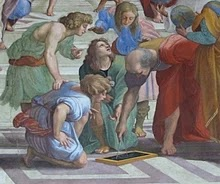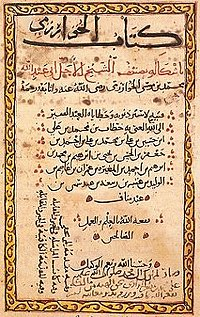## Pages

### Mathematics

Mathematics includes the study of such topics as quantity, structure, space, and changeMathematicians seek and use patterns to formulate new conjectures; they resolve the truth or falsity of conjectures by mathematical proof. When mathematical structures are good models of real phenomena, then mathematical reasoning can provide insight or predictions about nature. Through the use of abstraction and logic, mathematics developed from counting, calculation, measurement, and the systematic study of the shapes and motions of physical objects. Practical mathematics has been a human activity from as far back as written records exist. The research required to solve mathematical problems can take years or even centuries of sustained inquiry.

Rigorous arguments first appeared in Greek mathematics, most notably in Euclid's Elements. Since the pioneering work of Giuseppe Peano (1858–1932), David Hilbert (1862–1943), and others on axiomatic systems in the late 19th century, it has become customary to view mathematical research as establishing truth by rigorous deduction from appropriately chosen axioms and definitions. Mathematics developed at a relatively slow pace until the Renaissance, when mathematical innovations interacting with new scientific discoveries led to a rapid increase in the rate of mathematical discovery that has continued to the present day.

Mathematics is essential in many fields, including natural science, engineering, medicine, finance, and the social sciences. Applied mathematics has led to entirely new mathematical disciplines, such as statistics and game theory. Mathematicians engage in pure mathematics (mathematics for its own sake) without having any application in mind, but practical applications for what began as pure mathematics are often discovered later.

## History

The history of mathematics can be seen as an ever-increasing series of abstractions. The first abstraction, which is shared by many animals, was probably that of numbers: the realization that a collection of two apples and a collection of two oranges have something in common, namely quantity of their members.As evidenced by tallies found on bone, in addition to recognizing how to count physical objects, prehistoric peoples may have also recognized how to count abstract quantities, like time – days, seasons, years.

Evidence for more complex mathematics does not appear until around 3000 BC, when the Babylonians and Egyptians began using arithmetic, algebra and geometry for taxation and other financial calculations, for building and construction, and for astronomy. The most ancient mathematical texts from Mesopotamia and Egypt are from 2000–1800 BC. Many early texts mention Pythagorean triples and so, by inference, the Pythagorean theorem seems to be the most ancient and widespread mathematical development after basic arithmetic and geometry. It is in Babylonian mathematics that elementary arithmetic (addition, subtraction, multiplication and division) first appear in the archaeological record. The Babylonians also possessed a place-value system, and used a sexagesimal numeral system, still in use today for measuring angles and time.

Beginning in the 6th century BC with the Pythagoreans, the Ancient Greeks began a systematic study of mathematics as a subject in its own right with Greek mathematics. Around 300 BC, Euclid introduced the axiomatic method still used in mathematics today, consisting of definition, axiom, theorem, and proof. His textbook Elements is widely considered the most successful and influential textbook of all time.The greatest mathematician of antiquity is often held to be Archimedes (c. 287–212 BC) of Syracuse.He developed formulas for calculating the surface area and volume of solids of revolution and used the method of exhaustion to calculate the area under the arc of a parabola with the summation of an infinite series, in a manner not too dissimilar from modern calculus.Other notable achievements of Greek mathematics are conic sections (Apollonius of Perga, 3rd century BC),trigonometry (Hipparchus of Nicaea (2nd century BC),and the beginnings of algebra (Diophantus, 3rd century AD).

The Hindu–Arabic numeral system and the rules for the use of its operations, in use throughout the world today, evolved over the course of the first millennium AD in India and were transmitted to the Western world via Islamic mathematics. Other notable developments of Indian mathematics include the modern definition of sine and cosine, and an early form of infinite series.

During the Golden Age of Islam, especially during the 9th and 10th centuries, mathematics saw many important innovations building on Greek mathematics. The most notable achievement of Islamic mathematics was the development of algebra. Other notable achievements of the Islamic period are advances in spherical trigonometry and the addition of the decimal point to the Arabic numeral system. Many notable mathematicians from this period were Persian, such as Al-Khwarismi, Omar Khayyam and Sharaf al-Dīn al-Ṭūsī.

During the early modern period, mathematics began to develop at an accelerating pace in Western Europe. The development of calculus by Newton and Leibniz in the 17th century revolutionized mathematics. Leonhard Euler was the most notable mathematician of the 18th century, contributing numerous theorems and discoveries. Perhaps the foremost mathematician of the 19th century was the German mathematician Carl Friedrich Gauss, who made numerous contributions to fields such as algebra, analysis, differential geometry, matrix theory, number theory, and statistics. In the early 20th century, Kurt Gödel transformed mathematics by publishing his incompleteness theorems, which show that any axiomatic system that is consistent will contain unprovable propositions.

Mathematics has since been greatly extended, and there has been a fruitful interaction between mathematics and science, to the benefit of both. Mathematical discoveries continue to be made today. According to Mikhail B. Sevryuk, in the January 2006 issue of the Bulletin of the American Mathematical Society, "The number of papers and books included in the Mathematical Reviews database since 1940 (the first year of operation of MR) is now more than 1.9 million, and more than 75 thousand items are added to the database each year. The overwhelming majority of works in this ocean contain new mathematical theorems and their proofs."

### EtymologyThe word mathematics comes from Ancient Greek μάθημα (máthēma), meaning "that which is learnt","what one gets to know", hence also "study" and "science". The word for "mathematics" came to have the narrower and more technical meaning "mathematical study" even in Classical times. Its adjective is μαθηματικός (mathēmatikós), meaning "related to learning" or "studious", which likewise further came to mean "mathematical". In particular, μαθηματικὴ τέχνη (mathēmatikḗ tékhnē), Latin: ars mathematica, meant "the mathematical art".

Similarly, one of the two main schools of thought in Pythagoreanism was known as the mathēmatikoi (μαθηματικοί)—which at the time meant "teachers" rather than "mathematicians" in the modern sense.

In Latin, and in English until around 1700, the term mathematics more commonly meant "astrology" (or sometimes "astronomy") rather than "mathematics"; the meaning gradually changed to its present one from about 1500 to 1800. This has resulted in several mistranslations. For example, Saint Augustine's warning that Christians should beware of mathematici, meaning astrologers, is sometimes mistranslated as a condemnation of mathematicians.

The apparent plural form in English, like the French plural form les mathématiques (and the less commonly used singular derivative la mathématique), goes back to the Latin neuter plural mathematica (Cicero), based on the Greek plural τὰ μαθηματικά (ta mathēmatiká), used by Aristotle (384–322 BC), and meaning roughly "all things mathematical"; although it is plausible that English borrowed only the adjective mathematic(al) and formed the noun mathematics anew, after the pattern of physics and metaphysics, which were inherited from Greek. In English, the noun mathematics takes a singular verb. It is often shortened to maths or, in North America, math.

### Definitions of mathematics

Leonardo Fibonacci, the Italian mathematician who introduced the Hindu–Arabic numeral system invented between the 1st and 4th centuries by Indian mathematicians, to the Western World
Mathematics has no generally accepted definition. Aristotle defined mathematics as "the science of quantity", and this definition prevailed until the 18th century. Galileo Galilei (1564–1642) said, "The universe cannot be read until we have learned the language and become familiar with the characters in which it is written. It is written in mathematical language, and the letters are triangles, circles and other geometrical figures, without which means it is humanly impossible to comprehend a single word.

Without these, one is wandering about in a dark labyrinth." Carl Friedrich Gauss (1777–1855) referred to mathematics as "the Queen of the Sciences". Benjamin Peirce (1809–1880) called mathematics "the science that draws necessary conclusions". David Hilbert said of mathematics: "We are not speaking here of arbitrariness in any sense. Mathematics is not like a game whose tasks are determined by arbitrarily stipulated rules. Rather, it is a conceptual system possessing internal necessity that can only be so and by no means otherwise." Albert Einstein (1879–1955) stated that "as far as the laws of mathematics refer to reality, they are not certain; and as far as they are certain, they do not refer to reality."

Starting in the 19th century, when the study of mathematics increased in rigor and began to address abstract topics such as group theory and projective geometry, which have no clear-cut relation to quantity and measurement, mathematicians and philosophers began to propose a variety of new definitions. Some of these definitions emphasize the deductive character of much of mathematics, some emphasize its abstractness, some emphasize certain topics within mathematics. Today, no consensus on the definition of mathematics prevails, even among professionals. There is not even consensus on whether mathematics is an art or a science. A great many professional mathematicians take no interest in a definition of mathematics, or consider it undefinable. Some just say, "Mathematics is what mathematicians do."

Three leading types of definition of mathematics are called logicist, intuitionist, and formalist, each reflecting a different philosophical school of thought.All have severe problems, none has widespread acceptance, and no reconciliation seems possible.

An early definition of mathematics in terms of logic was Benjamin Peirce's "the science that draws necessary conclusions" (1870).In the Principia Mathematica, Bertrand Russell and Alfred North Whitehead advanced the philosophical program known as logicism, and attempted to prove that all mathematical concepts, statements, and principles can be defined and proved entirely in terms of symbolic logic. A logicist definition of mathematics is Russell's "All Mathematics is Symbolic Logic" (1903).

Intuitionist definitions, developing from the philosophy of mathematician L. E. J. Brouwer, identify mathematics with certain mental phenomena. An example of an intuitionist definition is "Mathematics is the mental activity which consists in carrying out constructs one after the other." A peculiarity of intuitionism is that it rejects some mathematical ideas considered valid according to other definitions. In particular, while other philosophies of mathematics allow objects that can be proved to exist even though they cannot be constructed, intuitionism allows only mathematical objects that one can actually construct.

Formalist definitions identify mathematics with its symbols and the rules for operating on them. Haskell Curry defined mathematics simply as "the science of formal systems".A formal system is a set of symbols, or tokens, and some rules telling how the tokens may be combined into formulas. In formal systems, the word axiom has a special meaning, different from the ordinary meaning of "a self-evident truth". In formal systems, an axiom is a combination of tokens that is included in a given formal system without needing to be derived using the rules of the system.